# Teaching Thermodynamics How do Mismatches between Chemistry and

• Slides: 48
Download presentationTeaching Thermodynamics: How do Mismatches between Chemistry and Physics Affect Student Learning? David E. Meltzer Department of Physics and Astronomy Iowa State University Ames, Iowa Supported in part by National Science Foundation grant DUE #9981140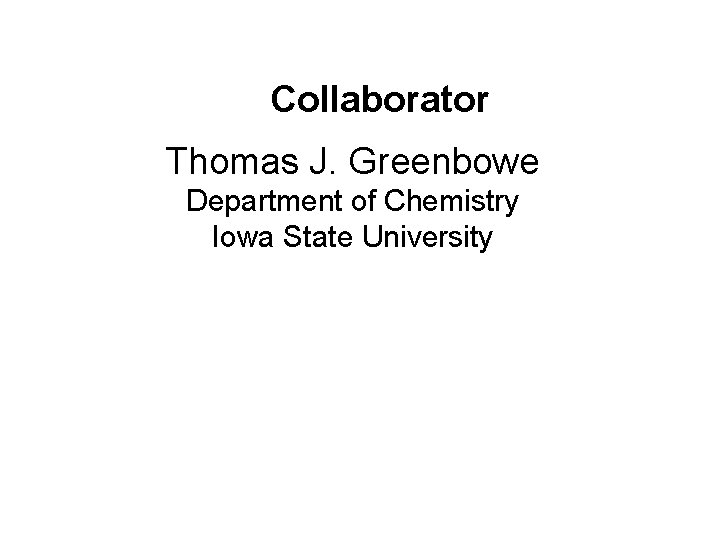Collaborator Thomas J. Greenbowe Department of Chemistry Iowa State UniversityOur Goal: Investigate learning difficulties in thermodynamics in both chemistry and physics courses • First focus on students’ initial exposure to thermodynamics (i. e. , in chemistry courses), then follow up with their next exposure (in physics courses). • Investigate learning of same or similar topics in two different contexts (often using different forms of representation). • Devise methods to directly address these learning difficulties. • Test materials with students in both courses; use insights gained in one field to inform instruction in the other.Outline 1. The physics/chemistry connection 2. First-semester chemistry: – state functions – heat, work, first law of thermodynamics 3. Second-semester physics: – heat, work, first law of thermodynamics – cyclic process 4. Second-semester chemistry: – second law of thermodynamics – Gibbs free energy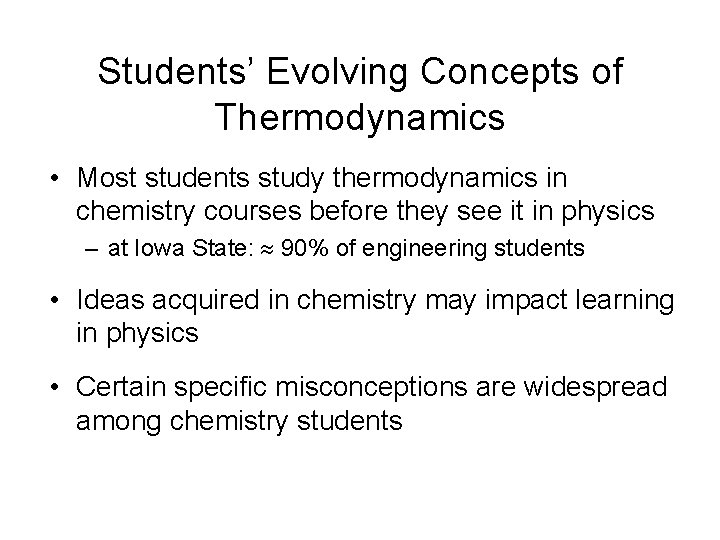Students’ Evolving Concepts of Thermodynamics • Most students study thermodynamics in chemistry courses before they see it in physics – at Iowa State: 90% of engineering students • Ideas acquired in chemistry may impact learning in physics • Certain specific misconceptions are widespread among chemistry students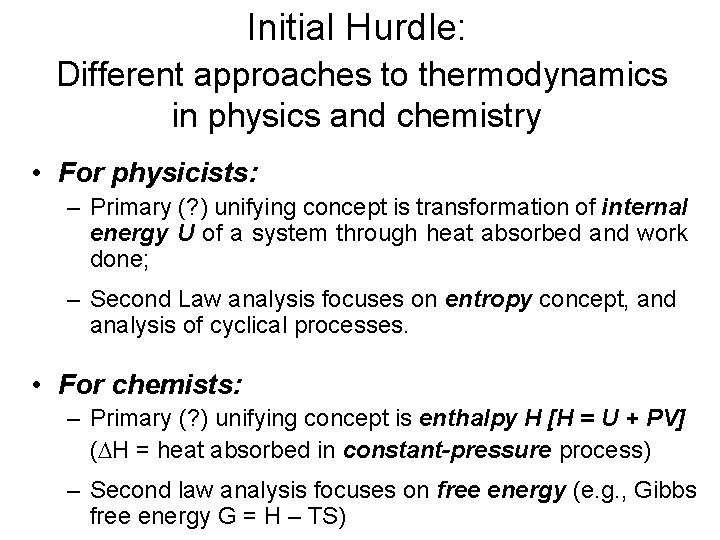Initial Hurdle: Different approaches to thermodynamics in physics and chemistry • For physicists: – Primary (? ) unifying concept is transformation of internal energy U of a system through heat absorbed and work done; – Second Law analysis focuses on entropy concept, and analysis of cyclical processes. • For chemists: – Primary (? ) unifying concept is enthalpy H [H = U + PV] ( H = heat absorbed in constant-pressure process) – Second law analysis focuses on free energy (e. g. , Gibbs free energy G = H – TS)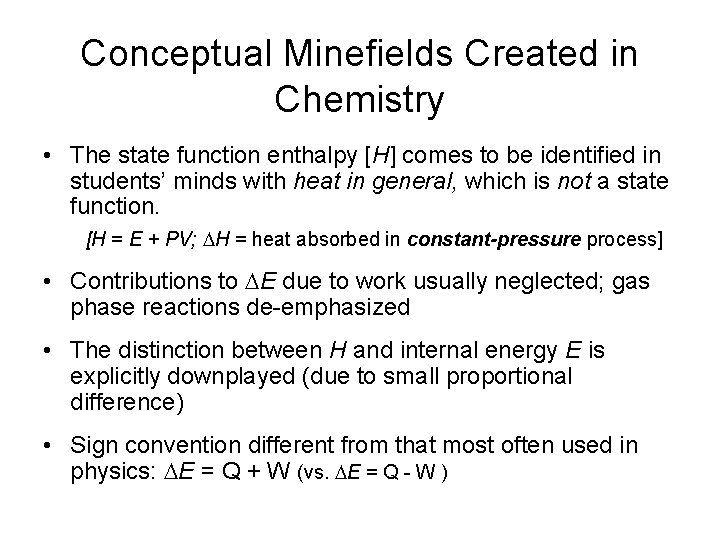Conceptual Minefields Created in Chemistry • The state function enthalpy [H] comes to be identified in students’ minds with heat in general, which is not a state function. [H = E + PV; H = heat absorbed in constant-pressure process] • Contributions to E due to work usually neglected; gas phase reactions de-emphasized • The distinction between H and internal energy E is explicitly downplayed (due to small proportional difference) • Sign convention different from that most often used in physics: E = Q + W (vs. E = Q - W )How might this affect physics instruction? • For many physics students, initial ideas about thermodynamics are formed during chemistry courses. • In chemistry courses, a particular state function (enthalpy) comes to be identified -- in students’ minds -- with heat in general, which is not a state function.Initial Objectives: Students’ understanding of “state functions” and First Law of Thermodynamics Diagnostic Strategy: Examine two different processes leading from state “A” to state “B”: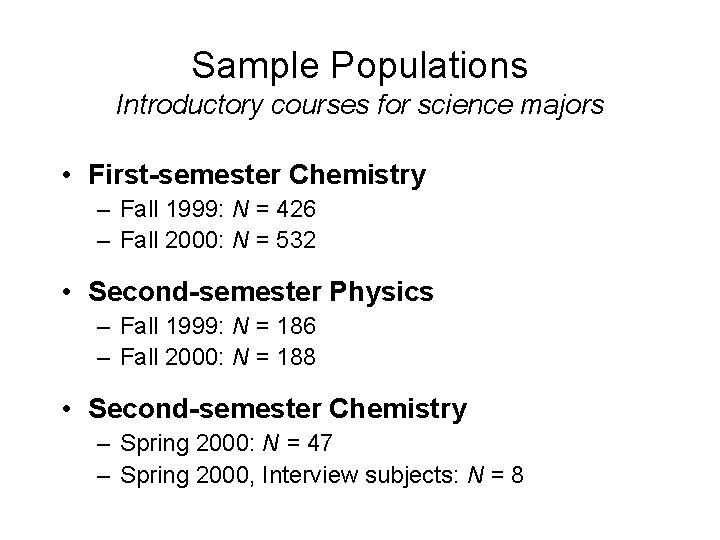Sample Populations Introductory courses for science majors • First-semester Chemistry – Fall 1999: N = 426 – Fall 2000: N = 532 • Second-semester Physics – Fall 1999: N = 186 – Fall 2000: N = 188 • Second-semester Chemistry – Spring 2000: N = 47 – Spring 2000, Interview subjects: N = 8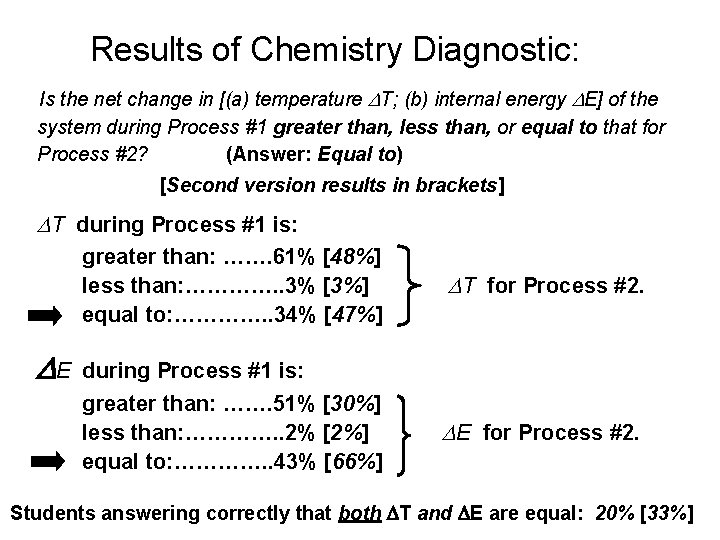Results of Chemistry Diagnostic: Is the net change in [(a) temperature T; (b) internal energy E] of the system during Process #1 greater than, less than, or equal to that for Process #2? (Answer: Equal to) [Second version results in brackets] T during Process #1 is: greater than: ……. 61% [48%] less than: …………. . 3% [3%] equal to: …………. . 34% [47%] E T for Process #2. during Process #1 is: greater than: ……. 51% [30%] less than: …………. . 2% [2%] equal to: …………. . 43% [66%] E for Process #2. Students answering correctly that both T and E are equal: 20% [33%]Results from Chemistry Diagnostic [Given in general chemistry course for science majors, Fall 2000, N =532] • 65% of students recognized that change in internal energy was same for both processes. • Only 47% of students recognized that change in temperature must be the same for both processes (since initial and final states are identical).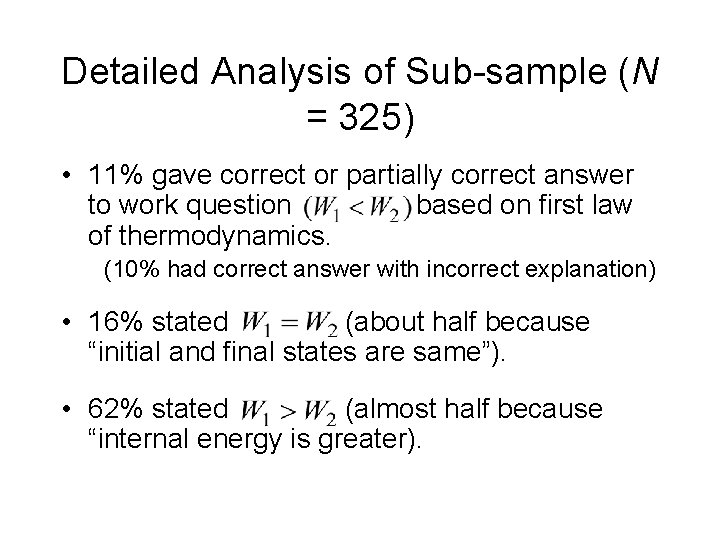Detailed Analysis of Sub-sample (N = 325) • 11% gave correct or partially correct answer to work question based on first law of thermodynamics. (10% had correct answer with incorrect explanation) • 16% stated (about half because “initial and final states are same”). • 62% stated (almost half because “internal energy is greater).Physics Diagnostic • Given in second semester of calculus-based introductory course. • Traditional course; thermal physics comprised 20% of course coverage. • Diagnostic administered in last week of course: – Fall 1999: practice quiz during last recitation; N = 186 – Fall 2000: practice quiz during final lecture; N = 188 – Spring 2001: practice quiz during last recitation; N = 279Samples of Students’ Answers (All considered correct) “ U = Q – W. For the same U, the system with more work done must have more Q input so process #1 is greater. ” “Q is greater for process 1 since Q = U + W and W is greater for process 1. ” “Q is greater for process one because it does more work, the energy to do this work comes from the Qin. ” “U = Q – W, Q = U + W, if U is the same and W is greater then Q is greater for Process #1. ”Students’ Reasoning on Work Question [Fall 2000: N = 188] • Correct or partially correct. . . 56% • Incorrect or missing explanation. . . . 14% • Work is independent of path. . 26% (majority explicitly assert path independence) • Other responses. . . . . 4%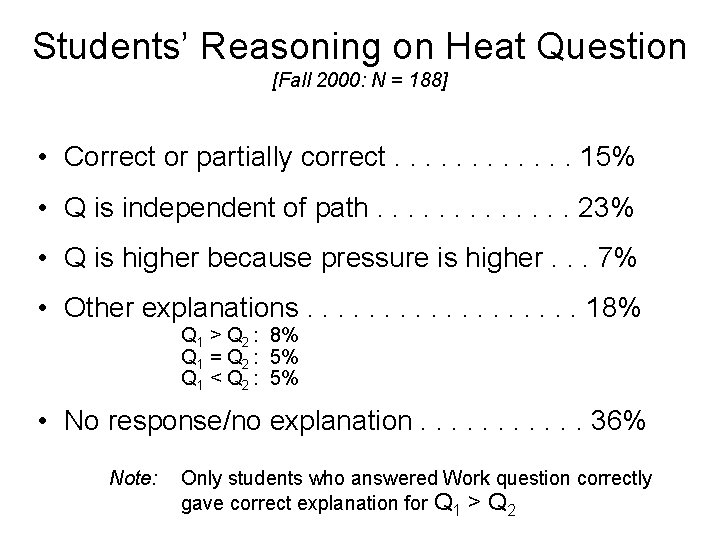Students’ Reasoning on Heat Question [Fall 2000: N = 188] • Correct or partially correct. . . 15% • Q is independent of path. . . 23% • Q is higher because pressure is higher. . . 7% • Other explanations. . . . 18% Q 1 > Q 2 : 8% Q 1 = Q 2 : 5% Q 1 < Q 2 : 5% • No response/no explanation. . . 36% Note: Only students who answered Work question correctly gave correct explanation for Q 1 > Q 2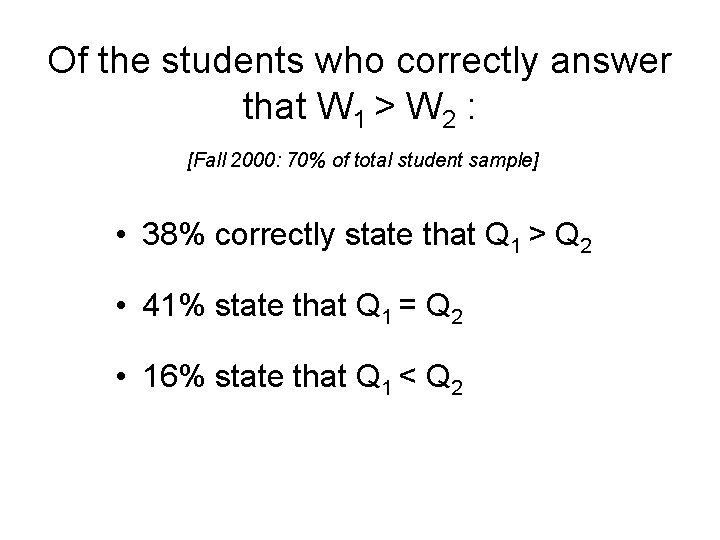Of the students who correctly answer that W 1 > W 2 : [Fall 2000: 70% of total student sample] • 38% correctly state that Q 1 > Q 2 • 41% state that Q 1 = Q 2 • 16% state that Q 1 < Q 2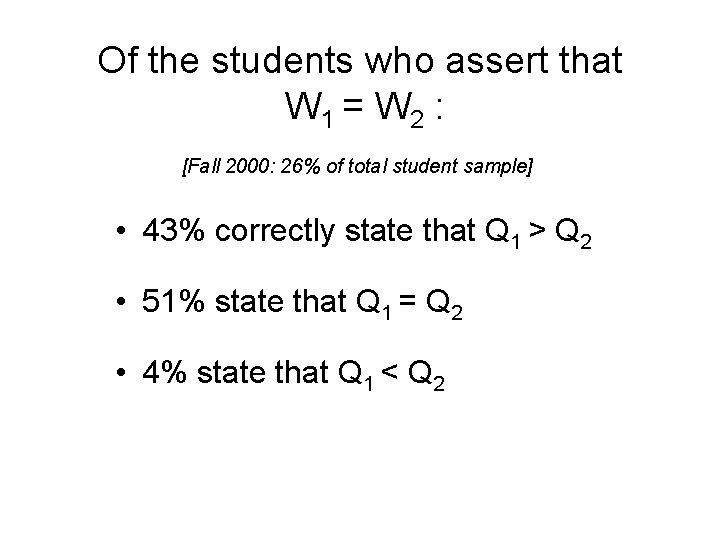Of the students who assert that W 1 = W 2 : [Fall 2000: 26% of total student sample] • 43% correctly state that Q 1 > Q 2 • 51% state that Q 1 = Q 2 • 4% state that Q 1 < Q 2Relation Between Answers on Work and Heat Questions • Probability of answering Q 1 > Q 2 is almost independent of answer to Work question. [However, correct explanations are only given by those who answer Work question correctly. ] • Probability of claiming Q 1 = Q 2 is slightly greater for those who answer W 1 = W 2. • Probability of justifying Q 1 = Q 2 by asserting that “Q is path-independent” is higher for those who answer Work question correctly. è Correct on Work question and state Q 1 = Q 2 : 61% claim “Q is path-independent” è Incorrect on Work question and state Q 1 = Q 2 : 37% claim “Q is path-independent”Conceptual Difficulties with Work • Difficulty interpreting work as “area under the curve” on a p-V diagram Only 50% able to give correct explanation for W 1 > W 2 • Belief that work done is independent of process About 15 -25% are under impression that work is (or behaves as) a state function.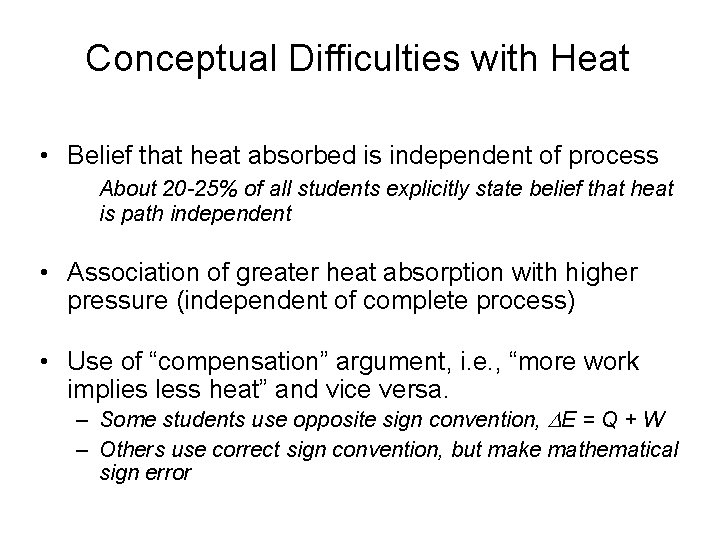Conceptual Difficulties with Heat • Belief that heat absorbed is independent of process About 20 -25% of all students explicitly state belief that heat is path independent • Association of greater heat absorption with higher pressure (independent of complete process) • Use of “compensation” argument, i. e. , “more work implies less heat” and vice versa. – Some students use opposite sign convention, E = Q + W – Others use correct sign convention, but make mathematical sign error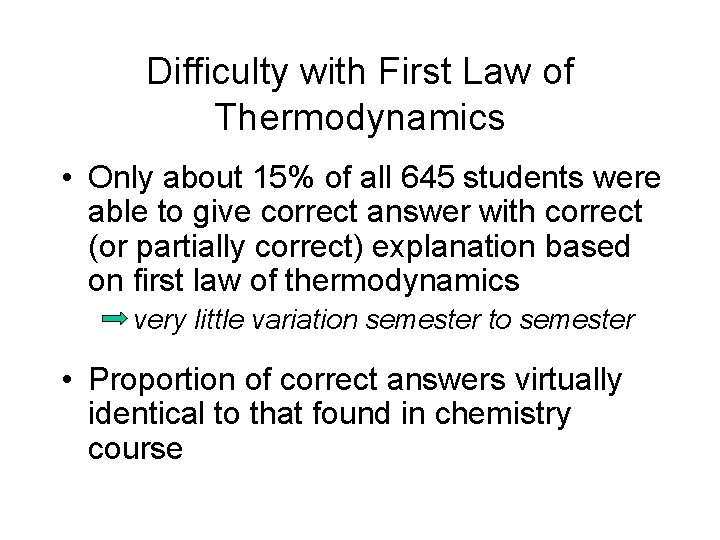Difficulty with First Law of Thermodynamics • Only about 15% of all 645 students were able to give correct answer with correct (or partially correct) explanation based on first law of thermodynamics very little variation semester to semester • Proportion of correct answers virtually identical to that found in chemistry course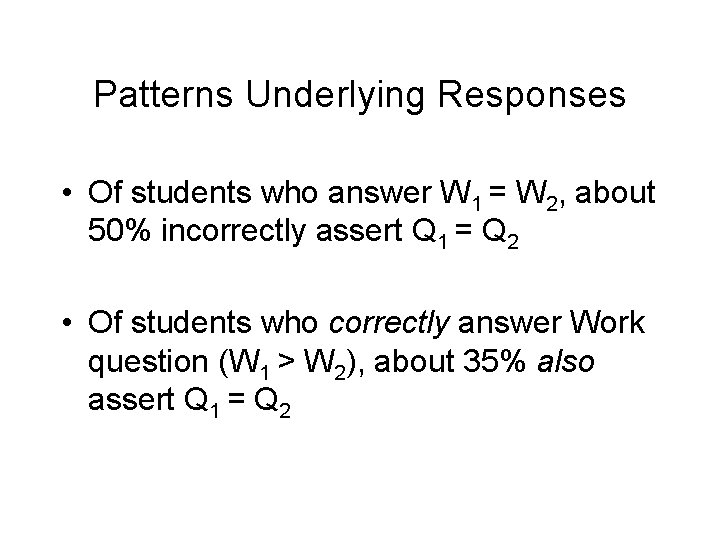Patterns Underlying Responses • Of students who answer W 1 = W 2, about 50% incorrectly assert Q 1 = Q 2 • Of students who correctly answer Work question (W 1 > W 2), about 35% also assert Q 1 = Q 2Justifications Given by Students Who Incorrectly Assert Q 1 = Q 2 • Students who answered Work question correctly usually claim “heat is independent of path” • Students who answered Work question incorrectly usually do not claim “heat is independent of path”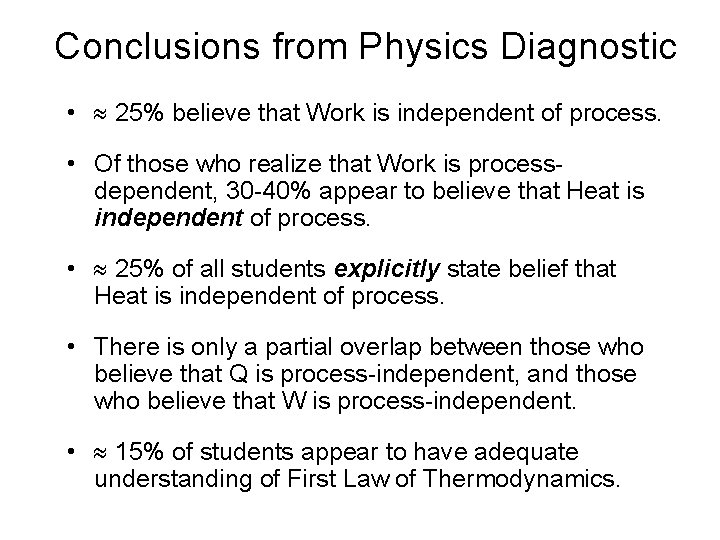Conclusions from Physics Diagnostic • 25% believe that Work is independent of process. • Of those who realize that Work is processdependent, 30 -40% appear to believe that Heat is independent of process. • 25% of all students explicitly state belief that Heat is independent of process. • There is only a partial overlap between those who believe that Q is process-independent, and those who believe that W is process-independent. • 15% of students appear to have adequate understanding of First Law of Thermodynamics.Conjectures Regarding Dynamics of Student Reasoning • Belief that heat is process-independent may not be strongly affected by realization that work is not process-independent. • Understanding process-dependence of work may strengthen mistaken belief that heat is independent of process.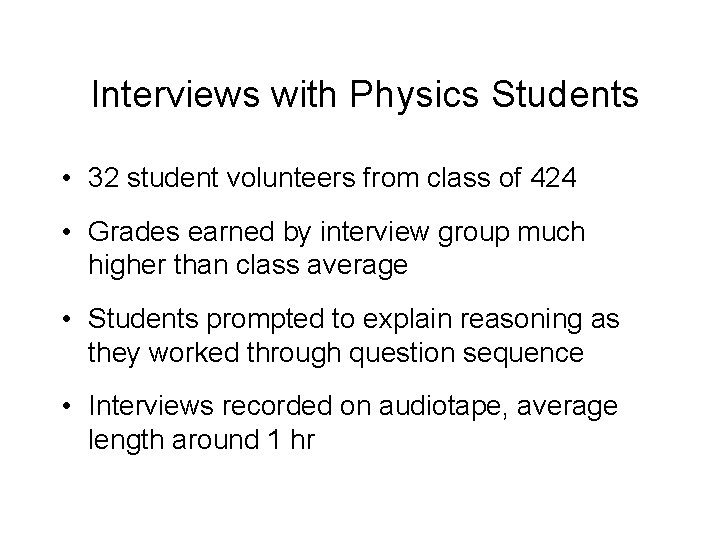Interviews with Physics Students • 32 student volunteers from class of 424 • Grades earned by interview group much higher than class average • Students prompted to explain reasoning as they worked through question sequence • Interviews recorded on audiotape, average length around 1 hrResults of Interviews • Very consistent with results of written diagnostics • Additional conceptual difficulties revealed • Yielded additional clues to explain students’ learning difficulties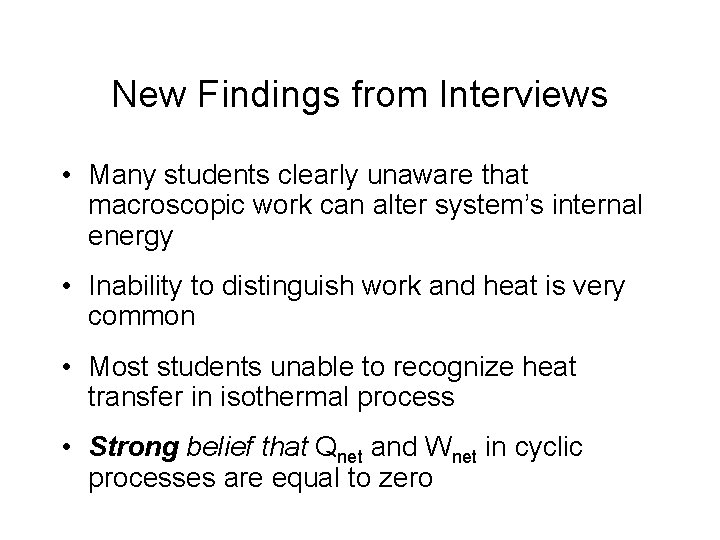New Findings from Interviews • Many students clearly unaware that macroscopic work can alter system’s internal energy • Inability to distinguish work and heat is very common • Most students unable to recognize heat transfer in isothermal process • Strong belief that Qnet and Wnet in cyclic processes are equal to zeroSummary of Results on First Law • No more than 15% of students are able to make effective use of first law of thermodynamics after introductory chemistry or introductory physics course. • Although similar errors regarding thermodynamics appear in thinking of both chemistry and physics students, possible linking of incorrect thinking needs further study.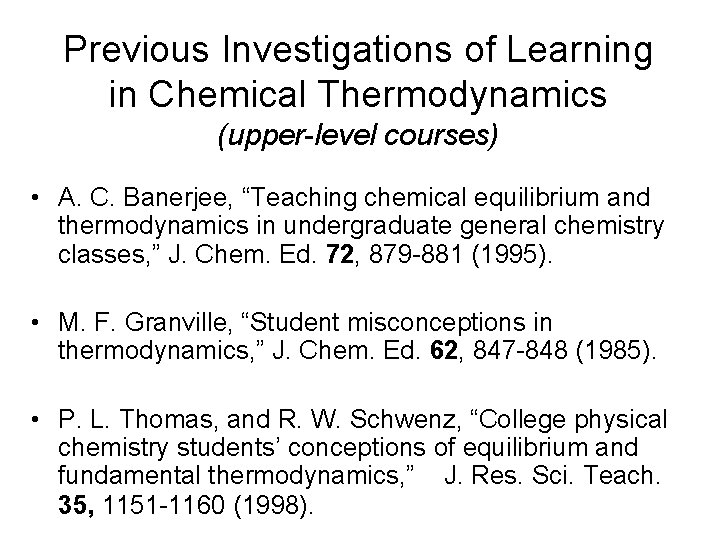Previous Investigations of Learning in Chemical Thermodynamics (upper-level courses) • A. C. Banerjee, “Teaching chemical equilibrium and thermodynamics in undergraduate general chemistry classes, ” J. Chem. Ed. 72, 879 -881 (1995). • M. F. Granville, “Student misconceptions in thermodynamics, ” J. Chem. Ed. 62, 847 -848 (1985). • P. L. Thomas, and R. W. Schwenz, “College physical chemistry students’ conceptions of equilibrium and fundamental thermodynamics, ” J. Res. Sci. Teach. 35, 1151 -1160 (1998).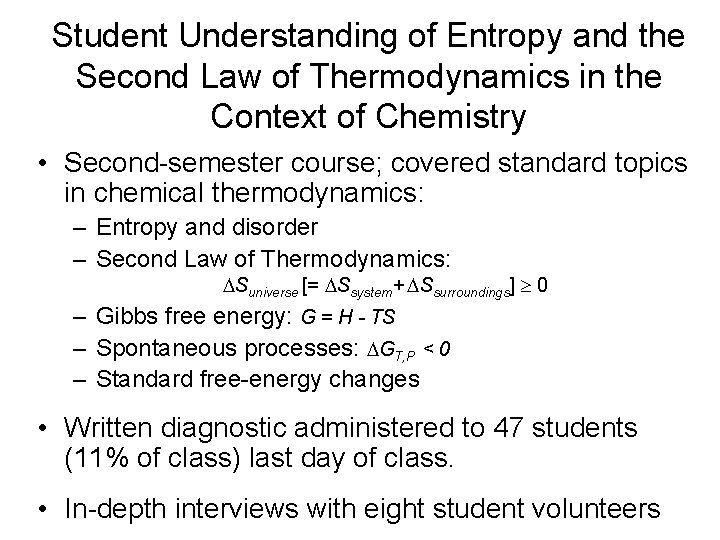Student Understanding of Entropy and the Second Law of Thermodynamics in the Context of Chemistry • Second-semester course; covered standard topics in chemical thermodynamics: – Entropy and disorder – Second Law of Thermodynamics: Suniverse [= Ssystem+ Ssurroundings] 0 – Gibbs free energy: G = H - TS – Spontaneous processes: GT, P < 0 – Standard free-energy changes • Written diagnostic administered to 47 students (11% of class) last day of class. • In-depth interviews with eight student volunteers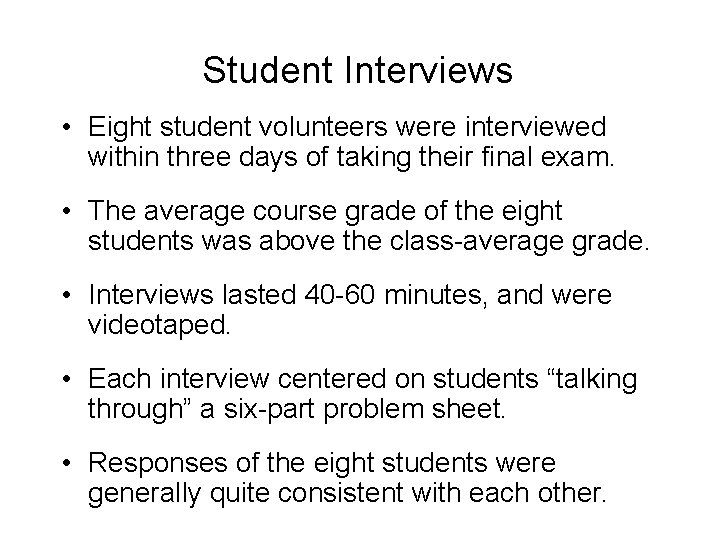Student Interviews • Eight student volunteers were interviewed within three days of taking their final exam. • The average course grade of the eight students was above the class-average grade. • Interviews lasted 40 -60 minutes, and were videotaped. • Each interview centered on students “talking through” a six-part problem sheet. • Responses of the eight students were generally quite consistent with each other.Students’ Guiding Conceptions (what they “know”) • H is equal to the heat absorbed by the system. • “Entropy” is synonymous with “disorder” • Spontaneous processes are characterized by increasing entropy • G = H - T S • G must be negative for a spontaneous process.Difficulties Interpreting Meaning of “ G” • Students seem unaware or unclear about the definition of G (i. e. , G = Gfinal – Ginitial) • Students often do not interpret “ G < 0” as meaning “G is decreasing” • The expression “ G” is frequently confused with “G” – “ G < 0” is interpreted as “G is negative, ” therefore, conclusion is that “G must be negative for a spontaneous process”Examples from Interviews Q: Tell me again the relationship between G and “spontaneous”? Student #7: I guess I don’t know, necessarily, about G; I know G. Q: Tell me what you remember about G. Student #7: I remember calculating it, and then if it was negative then it was spontaneous, if it was positive, being nonspontaneous. Q: What does that tell you about G itself. Suppose G is negative, what would be happening to G itself? Student #7: I don’t know because I don’t remember the relationship.Student Conception: If the process is spontaneous, G must be negative. Student #1: If it’s spontaneous, G would be negative. . . But if it wasn’t going to happen spontaneously, G would be positive. At equilibrium, G would be zero. . . if G doesn’t become negative, then it’s not spontaneous. As long as it stays in positive values, it can decrease, but [still be spontaneous]. Student #4: Say that the Gibbs free energy for the system before this process happened. . . was a negative number. . . [then] it can still increase and be spontaneous because it’s still going to be a negative number as long as it’s increasing until it gets to zero.Students’ confusion: apparently conflicting criteria for spontaneity • GT, P < 0 criterion, and equation G = H T S, refer only to properties of the system; • Suniverse > 0 refers to properties outside the system; Consequently, students are continually confused as to what is the “system” and what is the “universe, ” and which one determines the criteria for spontaneity.Student #2: I assume that S [in the equation G = H - T S] is the total entropy of the system and the surroundings. Student #3: “. . . I was just trying to recall whether or not the surroundings have an effect on whether or not it’s spontaneous. ” Student #6: “I don’t remember if both the system and surroundings have to be going generally up. . . I don’t know what effect the surroundings have on it. ”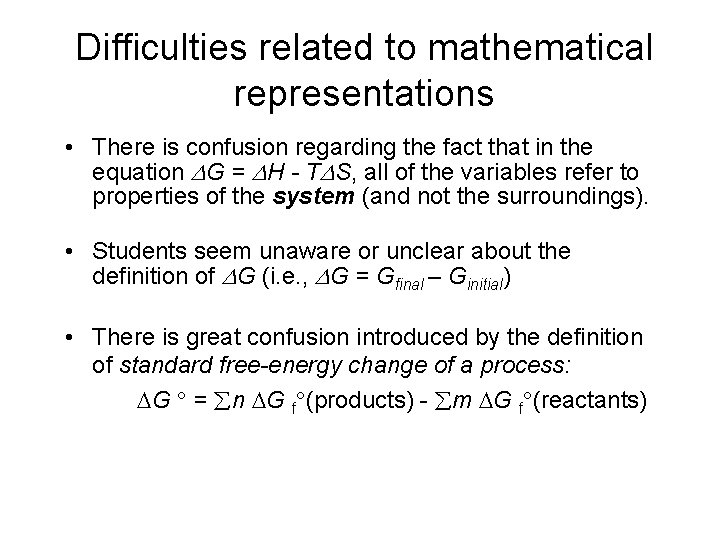Difficulties related to mathematical representations • There is confusion regarding the fact that in the equation G = H - T S, all of the variables refer to properties of the system (and not the surroundings). • Students seem unaware or unclear about the definition of G (i. e. , G = Gfinal – Ginitial) • There is great confusion introduced by the definition of standard free-energy change of a process: G = n G f (products) - m G f (reactants)Lack of awareness of constraints and conditions • There is little recognition that H equals heat absorbed only for constant-pressure processes • There appears to be no awareness that the requirement that G < 0 for a spontaneous process only holds for constant-pressure, constant-temperature processes.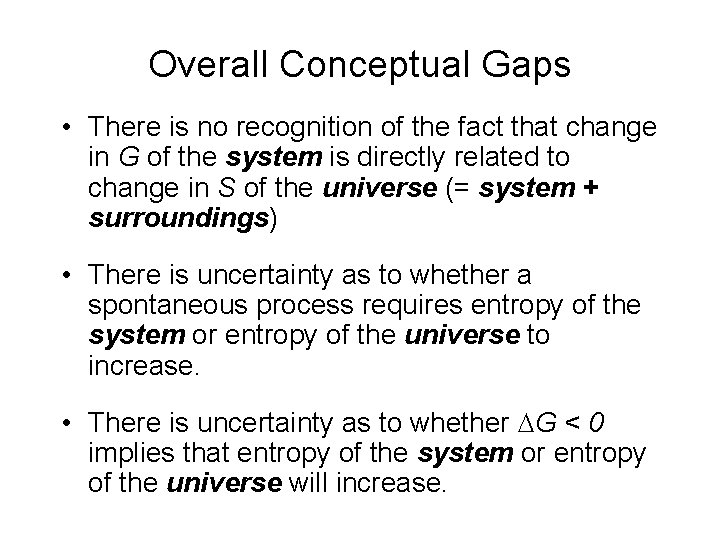Overall Conceptual Gaps • There is no recognition of the fact that change in G of the system is directly related to change in S of the universe (= system + surroundings) • There is uncertainty as to whether a spontaneous process requires entropy of the system or entropy of the universe to increase. • There is uncertainty as to whether G < 0 implies that entropy of the system or entropy of the universe will increase.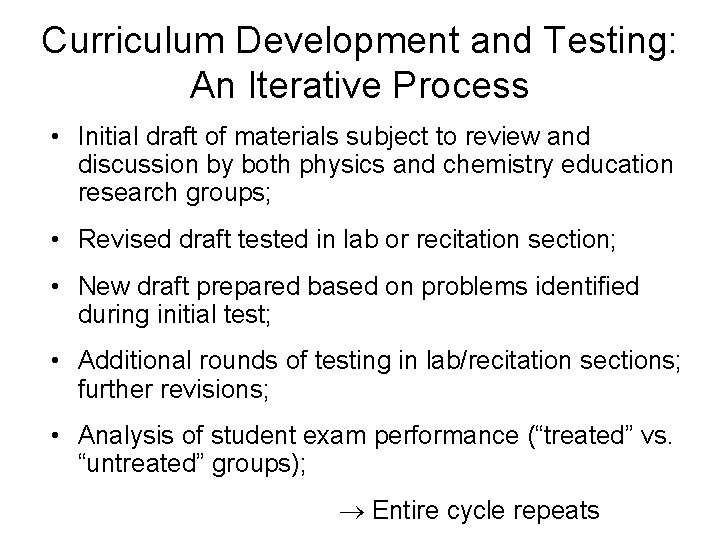Curriculum Development and Testing: An Iterative Process • Initial draft of materials subject to review and discussion by both physics and chemistry education research groups; • Revised draft tested in lab or recitation section; • New draft prepared based on problems identified during initial test; • Additional rounds of testing in lab/recitation sections; further revisions; • Analysis of student exam performance (“treated” vs. “untreated” groups); Entire cycle repeats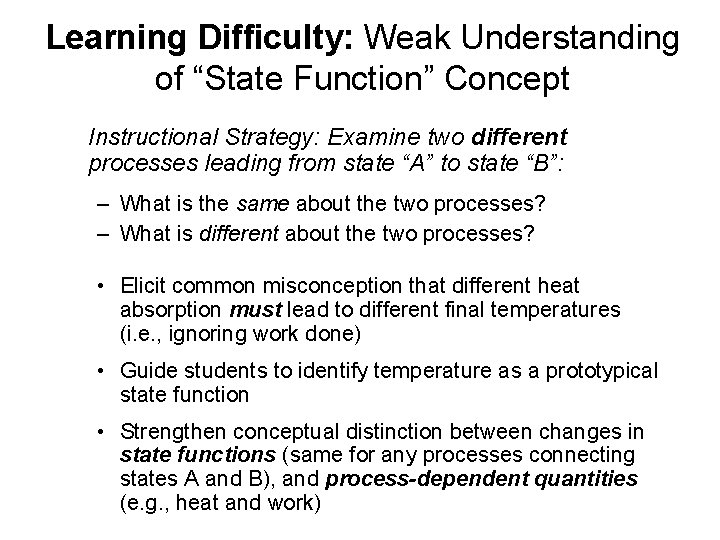Learning Difficulty: Weak Understanding of “State Function” Concept Instructional Strategy: Examine two different processes leading from state “A” to state “B”: – What is the same about the two processes? – What is different about the two processes? • Elicit common misconception that different heat absorption must lead to different final temperatures (i. e. , ignoring work done) • Guide students to identify temperature as a prototypical state function • Strengthen conceptual distinction between changes in state functions (same for any processes connecting states A and B), and process-dependent quantities (e. g. , heat and work)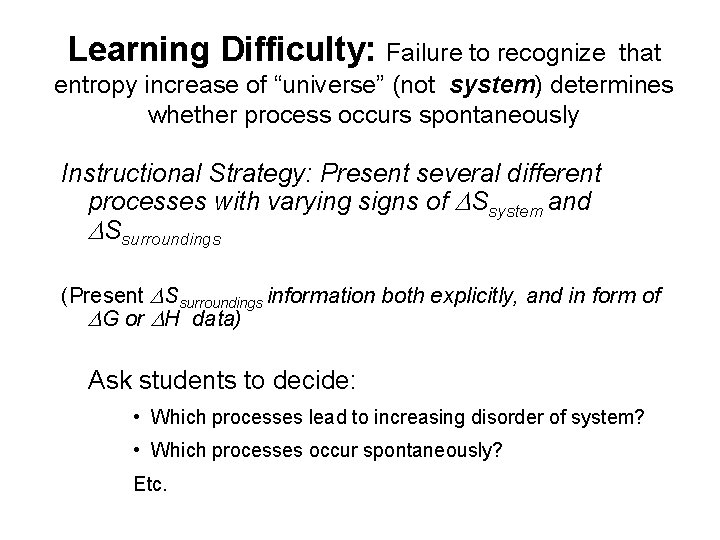Learning Difficulty: Failure to recognize that entropy increase of “universe” (not system) determines whether process occurs spontaneously Instructional Strategy: Present several different processes with varying signs of Ssystem and Ssurroundings (Present Ssurroundings information both explicitly, and in form of G or H data) Ask students to decide: • Which processes lead to increasing disorder of system? • Which processes occur spontaneously? Etc.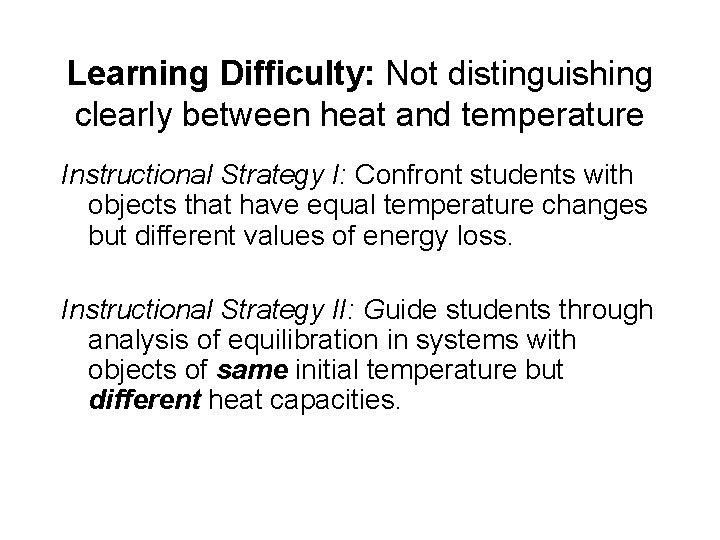Learning Difficulty: Not distinguishing clearly between heat and temperature Instructional Strategy I: Confront students with objects that have equal temperature changes but different values of energy loss. Instructional Strategy II: Guide students through analysis of equilibration in systems with objects of same initial temperature but different heat capacities.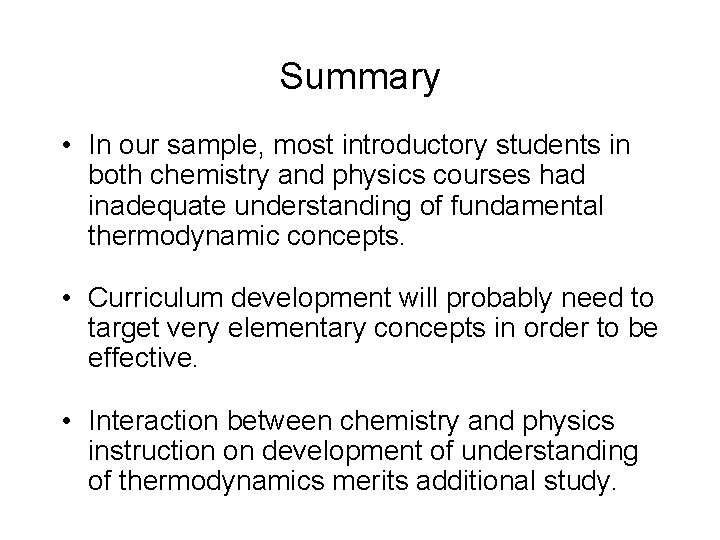Summary • In our sample, most introductory students in both chemistry and physics courses had inadequate understanding of fundamental thermodynamic concepts. • Curriculum development will probably need to target very elementary concepts in order to be effective. • Interaction between chemistry and physics instruction on development of understanding of thermodynamics merits additional study.